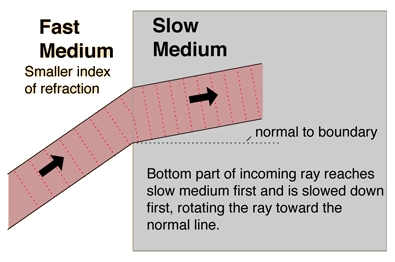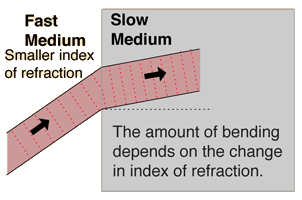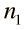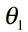# Refraction of Light

Refraction is the bending of a wave when it enters a medium where its speed is different. The refraction of light when it passes from a fast medium to a slow medium bends the light ray toward the normal to the boundary between the two media. The amount of bending depends on the indices of refraction of the two media and is described quantitatively by Snell's Law.

 Refraction is responsible for image formation by lenses and the eye.The bending of refraction can be visualized in terms of Huygens' principle. As the speed of light is reduced in the slower medium, the wavelength is shortened proportionately. The frequency is unchanged; it is a characteristic of the source of the light and unaffected by medium changes.

 Refraction and the eye Refraction of sound
 Refraction of light by water Refraction and focal length
Index

Lens concepts

 HyperPhysics***** Light and Vision R Nave
Go Back

# Index of Refraction

The index of refraction is defined as the speed of light in vacuum divided by the speed of light in the medium.The indices of refraction of some common substances are given below with a more complete description of the indices for optical glasses given elsewhere. The values given are approximate and do not account for the small variation of index with light wavelength which is called dispersion.

 Material n Material n Vacuum 1.000 Ethyl alcohol 1.362 Air 1.000277 Glycerine 1.473 Water 4/3 Ice 1.31 Carbon disulfide 1.63 Polystyrene 1.59 Methylene iodide 1.74 Crown glass 1.50-1.62 Diamond 2.417 Flint glass 1.57-1.75

 Refraction and the eye Refraction of sound
 Table of refractive indices
Index

Lens concepts

 HyperPhysics***** Light and Vision R Nave
Go Back

# Snell's Law

Snell's Law relates the indices of refraction n of the two media to the directions of propagation in terms of the angles to the normal. Snell's law can be derived from Fermat's Principle or from the Fresnel Equations.Enter data below, then click the symbol of the quantity you wish to calculate.

Indices of refraction:==

Angles with surface normal:= °= °

Enter data and then click on the symbol for the quantity you wish to calculate in the active equation above. The numbers will not be forced to be consistent until you click on the quantity to calculate. Indices of refraction must be greater than or equal to 1, so values less than 1 do not represent a physically possible system.

If the incident medium has the larger index of refraction, then the angle with the normal is increased by refraction. The larger index medium is commonly called the "internal" medium, since air with n=1 is usually the surrounding or "external" medium. You can calculate the condition for total internal reflection by setting the refracted angle = 90° and calculating the incident angle. Since you can't refract the light by more than 90°, all of it will reflect for angles of incidence greater than the angle which gives refraction at 90°.

Index

Lens concepts

 HyperPhysics***** Light and Vision R Nave
Go Back